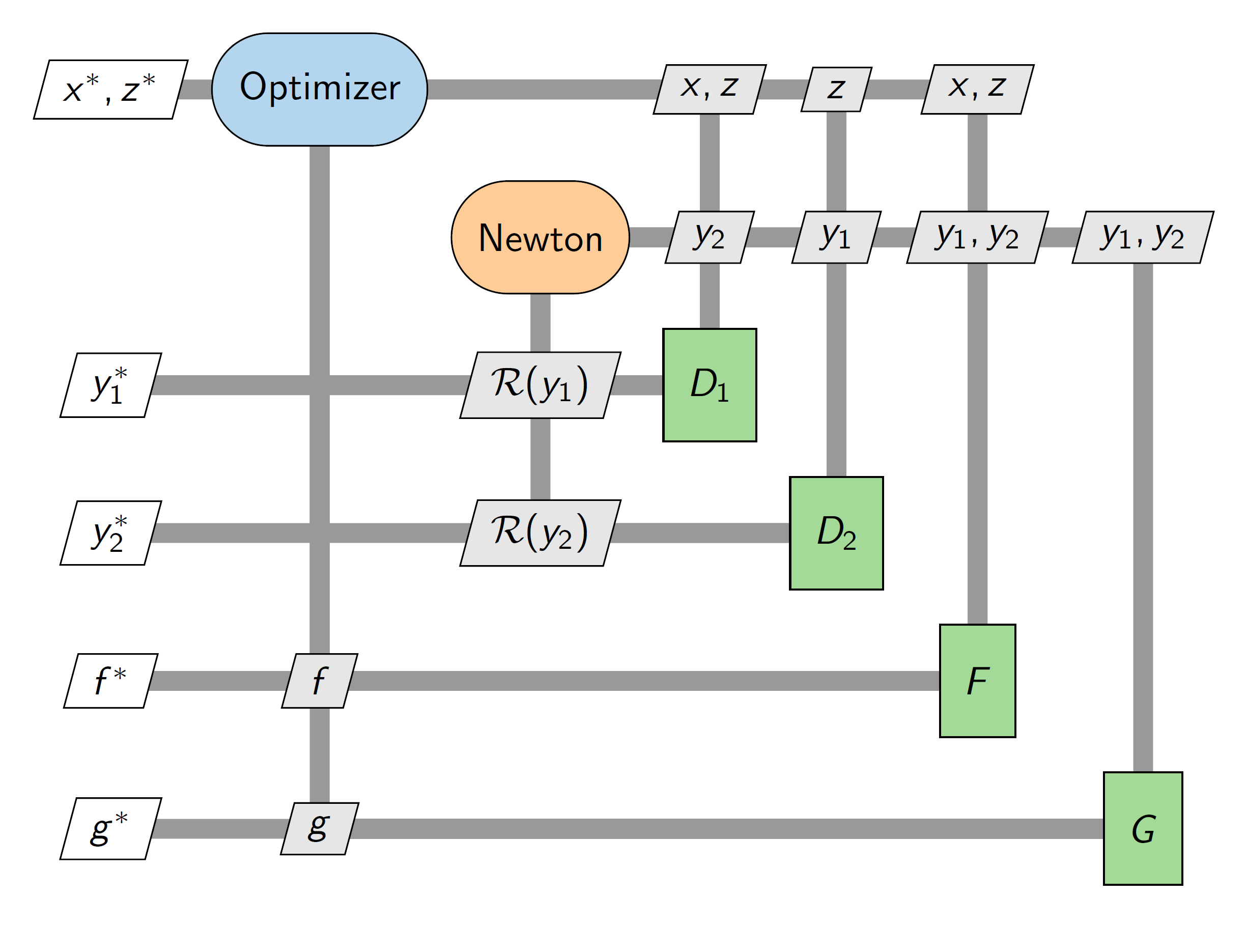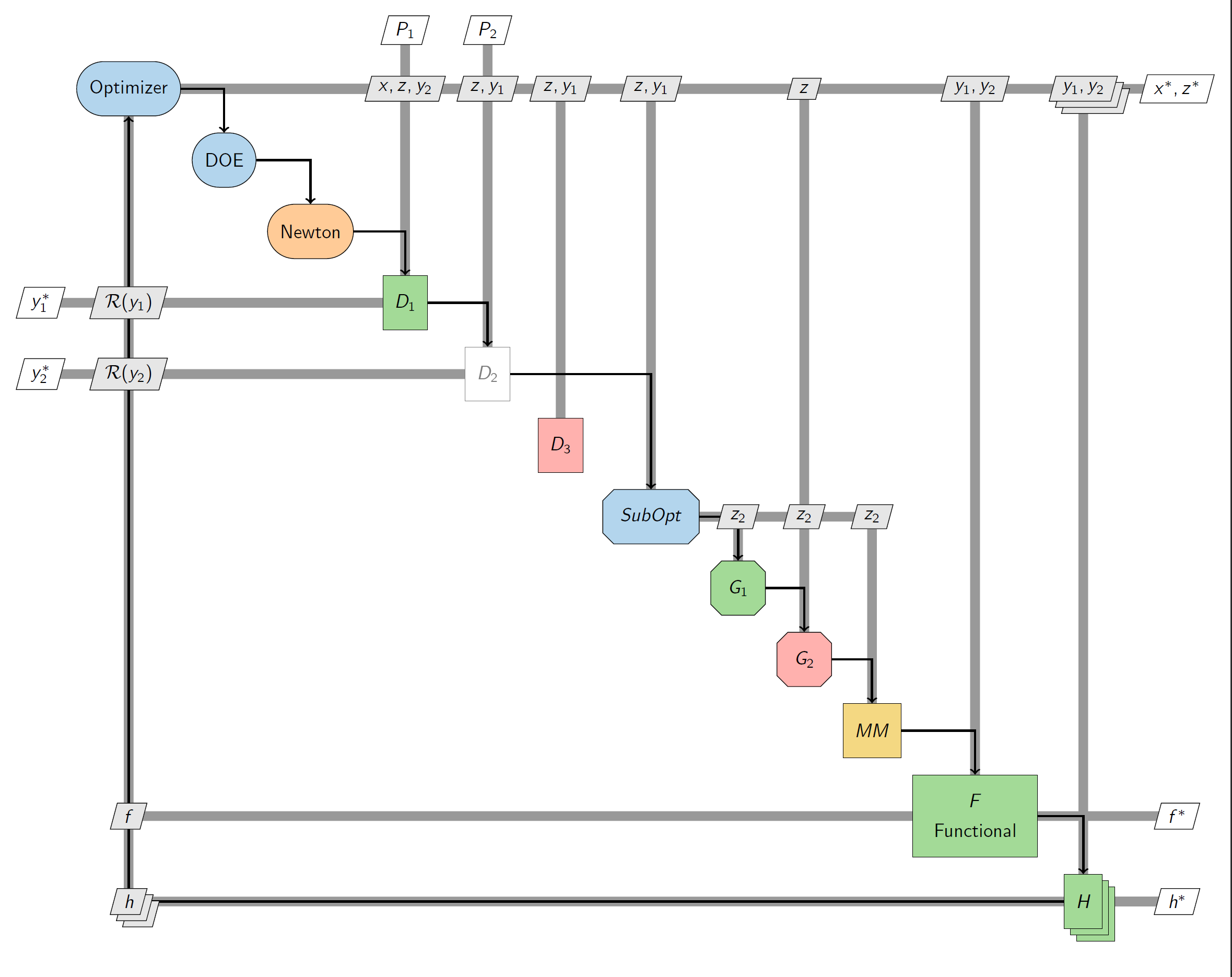# Examples

Here is a simple example. There are some other more advanced things you can do as well. Check out the examples folder for more complex scripts.

from pyxdsm.XDSM import XDSM, OPT, SOLVER, FUNC, LEFT

# Change use_sfmath to False to use computer modern
x = XDSM(use_sfmath=True)

x.connect("opt", "D1", "x, z")
x.connect("opt", "D2", "z")
x.connect("opt", "F", "x, z")
x.connect("solver", "D1", "y_2")
x.connect("solver", "D2", "y_1")
x.connect("D1", "solver", r"\mathcal{R}(y_1)")
x.connect("solver", "F", "y_1, y_2")
x.connect("D2", "solver", r"\mathcal{R}(y_2)")
x.connect("solver", "G", "y_1, y_2")

x.connect("F", "opt", "f")
x.connect("G", "opt", "g")

x.write("mdf")


This will output mdf.tex, a standalone tex document that (by default) is also compiled to mdf.pdf, shown below:## More complicated example

Here is an example that uses a whole bunch of the more advanced features in pyXDSM.It is mostly just a reference for all the customizations you can do. The code for this diagram is provided here